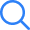# NCERT Solutions Class 12 Maths Chapter 5 Exercise 5.4

NCERT Solutions Class 12 Maths Chapter 5 Continuity and Differentiability Exercise 5.4 is developed by Selfstudys subject experts. They have put their expertise in solving every single question of 5.4 so that you can better understand the problem and be able to solve them by yourself. If you want to understand Maths lesson 1 thoroughly you need to solve their problems fully. In case, while solving the problems you face any difficulty you can seek help from the NCERT Solutions Class 12 Maths Chapter 5 Exercise 5.4.

Maths Chapter 5 is based on the topics that are crucial for students to enhance their knowledge in the subject. Furthermore, the solved problems of Exercise 5.4 guide students to get step wise solutions to each problem precisely.

## Advantages of NCERT Solutions Class 12 Maths Chapter 5 Exercise 5.4

Solving NCERT Class 12 Maths Chapter 5 Exercise 5.4 has very different advantages. For instance, solving questions will give you a clear understanding of the chapter and enable you to answer the difficulty level of questions too. Further, using the solutions will help you to learn the methodology which you can use in board exams to answer the questions. Also, while solving the Exercise 5.4 problem, if you see any concept that is new for you then you can use NCERT solutions to understand the core concepts behind those questions.

NCERT Class 12 Maths Solutions Chapter 5 Exercise 5.4 aid you in grasping the concepts more precisely and deeply. It further helps you to prepare for the maths exam and competitive exams too, because it is developed as per the syllabus of CBSE.

Selfstudys Exercise 5.4 Maths Class 12 Chapter 5 does not only help you to answer your questions but also gives you some tests to let you know how much more attention is required to master this lesson.

## Refer To The NCERT Solutions Class 12 Maths Chapter 5 Exercise 5.4

If you have forgotten about the formulas and understanding of the concepts in Chapter 5, you can refer to the NCERT Solutions Class 12 Maths Chapter 5 Exercise 5.4. It will assist you to comprehend the topics and appropriate formulas to answer the questions. Also many questions are there in Chapter 5 that are important from the exam perspective, so you can solve them as well. It will increase your chances to score more marks in the final board exams.

Exercise 5.4 is full of questions that need to be solved by students to practice them. Also, the subject experts have curated them to keep things easier to understand.

### Features of NCERT Solutions Chapter 5 Exercise 5.4

Every single chapter contains a lot of questions, however the expert team has the responsibility to prepare them in a way that doesn't feel overwhelming to the students. Below are a few features of Exercise 5.4 solutions PDF -

1. All answers are created in a very simple and easy to understand manner.
2. Answers are available in a stepwise manner.
3. Clear presentation of the questions and their answers are available in the PDF of Exercise 5.4.×
Open Now